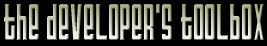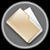This section of the archives stores flipcode's complete Developer Toolbox collection, featuring a variety of mini-articles and source code contributions from our readers.OpenGL Camera   Submitted byAs there are so many questions about making a camera on different boards I decided to take my camera and stuff it into a small and easy class. It doesnt contain any math, because theres no need to use slow trig functions, when your 3D-API already has everything you need. This matrix camera is limited to 3 actions: setting up the view matrix, moving and rotating. As its using a normal transformation matrix this code can also be used for all game objects like planes, cars and whatever you want. Thats the reason why there are always two versions. One local and one global, so an explosion can knock your car to the north while its rotating around its own axis. If need be I can provide the same camera for use with DirectX or a more complex version, which will do the culling for you and encapsulates the perspective setup.

To use this camera, simply add camera.cpp to your project and include camera.h wherever you need it. A few examples how to use the camera class.

Creating a camera can be done in a few ways.

Camera Cam; //Position will be set to (0,0,0)
Camera Cam(x,y,z); //Position will be set to (x,y,z) To place the camera somewhere else you can:
Cam.Position=newX;
Cam.Position=newY;
Cam.Position=newZ; In your render function before drawing anything you have to call Cam.setView(); To rotate around the (global) y-axis you call Cam.rotateGlob(degree, 0,1,0); while to look up/down you would call Cam.rotateLoc(degree, 1,0,0); Moving forward would be Cam.moveLoc(0,0,distance); while being shoved in a fixed direction would be Cam.moveGlob(deltaX, deltaY, deltaZ); of if you have a direction vector and a distance you call Cam.moveGlobal(dirX, dirY,dirZ, distance); A more complete version is available if you like, which also handles the perspective matrix and has functions to cull spheres, points and boxes.

Currently browsing [openglcamera.zip] (2,929 bytes) - [camera.cpp] - (3,215 bytes)

 ```#include "camera.h"/* The constructor will set the matrix to identity, except the inverted z-axis. I cant get used to "forward" being negative, but if this bothers you feel free to change the marked parts.Also, if you dont like to access the vectors like float-arrays you can always use vector-pointers, as long as they only contain three floats like this: struct vector{float x,y,z;}; and replace the float* with vector* in the header file. */ Camera::Camera(float x, float y, float z) { memset(Transform, 0, 16*sizeof(float)); Transform = 1.0f; Transform = 1.0f; Transform = -1.0f; Transform = 1.0f; Transform = x; Transform = y; Transform = z; Right=&Transform; Up=&Transform; Forward=&Transform; Position=&Transform; }Camera::~Camera() {}/* This one does pretty much the same as gluLookAt, just that it doesnt require to extract the vectors for gluLookAt and have it rebuild the matrix we already got. */ void Camera::setView() { glMatrixMode(GL_MODELVIEW); glLoadIdentity(); float viewmatrix={//Remove the three - for non-inverted z-axis Transform, Transform, -Transform, 0, Transform, Transform, -Transform, 0, Transform, Transform, -Transform, 0, -(Transform*Transform + Transform*Transform + Transform*Transform), -(Transform*Transform + Transform*Transform + Transform*Transform), //add a - like above for non-inverted z-axis (Transform*Transform + Transform*Transform + Transform*Transform), 1}; glLoadMatrixf(viewmatrix); }void Camera::moveLoc(float x, float y, float z, float distance) { float dx=x*Transform + y*Transform + z*Transform; float dy=x*Transform + y*Transform + z*Transform; float dz=x*Transform + y*Transform + z*Transform; Transform += dx * distance; Transform += dy * distance; Transform += dz * distance; }void Camera::moveGlob(float x, float y, float z, float distance) { Transform += x * distance; Transform += y * distance; Transform += z * distance; }/* Here we let OpenGls (most likely quite optimized) functions do the work. Note that its transformations already are in local coords. */ void Camera::rotateLoc(float deg, float x, float y, float z) { glMatrixMode(GL_MODELVIEW); glPushMatrix(); glLoadMatrixf(Transform); glRotatef(deg, x,y,z); glGetFloatv(GL_MODELVIEW_MATRIX, Transform); glPopMatrix(); }/* We have to invert the rotations to get the global axes in local coords. Luckily thats just the transposed in this case. */ void Camera::rotateGlob(float deg, float x, float y, float z) { float dx=x*Transform + y*Transform + z*Transform; float dy=x*Transform + y*Transform + z*Transform; float dz=x*Transform + y*Transform + z*Transform; glMatrixMode(GL_MODELVIEW); glPushMatrix(); glLoadMatrixf(Transform); glRotatef(deg, dx,dy,dz); glGetFloatv(GL_MODELVIEW_MATRIX, Transform); glPopMatrix(); } ```

Currently browsing [openglcamera.zip] (2,929 bytes) - [camera.h] - (1,814 bytes)

 ```#ifndef CAMERA_H #define CAMERA_H/* All the Win/OpenGL stuff.. if youre not using Windows you most likely know what goes here */ #ifdef _WIN32 #define WIN32_LEAN_AND_MEAN #include #include #pragma comment (lib, "opengl32.lib") #pragma comment (lib, "glu32.lib") #endif/* Usage: You can access the Right/Up/Forward vectors like a float array, but cannot write to them, because that would screw up the matrix. Same goes for Position, except its safe to write to it.setView() has to be called before you draw anything. Just call it instead of gluLookAt (which most are using)move and rotate come in two versions. Loc means the transformation is in local coords, so rotating around (1,0,0) means youre rotating around your current Right-vector while Glob would rotate around the global x-axis.Most likely you will use Loc for controlling the camera, though Glob can be usefull if you need to apply physics. Also walking characters will usually rotate around the global rather than around their local Up, while flying objects will always use local axes.If talking about objects when this is a camera confuses you: if you drop the setView() method you can use this for objects in your world too. Just rename the class to Object3D or something and derive a camera class from it. */ class Camera { public: float const *Right, *Up, *Forward; float *Position; private: float Transform;public: Camera(float x=0.0f, float y=0.0f, float z=0.0f); ~Camera(); void setView(); void moveLoc(float x, float y, float z, float distance=1); void moveGlob(float x, float y, float z, float distance=1); void rotateLoc(float deg, float x, float y, float z); void rotateGlob(float deg, float x, float y, float z); };#endif ```

The zip file viewer built into the Developer Toolbox made use of the zlib library, as well as the zlibdll source additions.